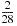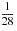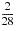# 15.10 - Static Row Partition Elimination - Teradata Database

## Teradata Database SQL Request and Transaction Processing

prodname
vrm_release
15.10
category
Programming Reference
User Guide
featnum
B035-1142-151K

Static row partition elimination, especially when applied at multiple levels, can significantly reduce the number of data blocks Teradata Database must read and process to respond to a query. Static row partition elimination is based on constant conditions on the partitioning columns and system‑derived columns PARTITION and PARTITION#Ln. The term constant conditions refers to literal values and predicate conditions such as equality, inequality, and BETWEEN and the substitution of any CURRENT_TIME, CURRENT_TIMESTAMP, or USING values for a specific plan and substitution of any DATE, CURRENT_DATE, TEMPORAL_DATE, or USER values.

Teradata Database applies static row partition elimination to each level independently, and combines the result into a single row partition elimination list. If the system cannot eliminate any row partitions at a level, then it must process all the row partitions for that level. The same algorithms as used for single‑level row partitioning are used at each level. The exception to this is conditions on system‑derived column PARTITION#Ln, which the row partition elimination algorithms consider for level n instead of conditions on system‑derived column PARTITION. The system considers conditions on both the system‑derived column PARTITION and the system‑derived column PARTITION#L1 for single‑level row partitioning.

Static partition elimination for a table or join index that has 8-byte partitioning is limited to 8,000 partitions, and no further static partition elimination occurs for that table or join index. Teradata Database applies static partition elimination to the first partitioning level, then the second partitioning level, and so on until either partition elimination completes or the limit of 8,000 non‑eliminated partitions is reached. If the limit is reached, the remaining partitions in that level and any lower levels are not eliminated.

If there are constant equality constraints on the partitioning columns of a partitioning expression, static row partition elimination occurs. All but the specified row partition for that partitioning expression is eliminated.

You should always run EXPLAIN request modifiers and measure actual system performance to verify that the desired partition elimination and performance occur for a candidate partitioning scheme and workload. If the results of your verification tests are not acceptable, or if they are not at the performance levels you expected, you should consider using a different partitioning scheme, or even using no partitioning, and then make other physical database design changes or query modifications such as adding constant conditions on the partitioning columns to obtain the level of performance you need and want.

If there are constant inequality constraints on the row partitioning columns of a row partitioning expression, static row partition elimination can occur when there is a single partitioning column for a RANGE_N partitioning expression and the test value expression is a recognized non‑decreasing linear expression based on the partitioning column. The recognized linear expressions are the following.

• A row partitioning column.
• A CAST, Teradata conversion, or EXTRACT of a recognized linear expression.
• The sum of 2 recognized linear expressions.
• The sum of a recognized linear expression and a constant expression.
• The sum of a constant expression and a recognized linear expression.
• The difference of a recognized linear expression and a constant expression.
• The product of a row partitioning column and a constant expression, where the value of the constant expression is greater than 0.
• The product of a constant expression and a row partitioning column, where the value of the constant expression is greater than 0.
• The division of a row partitioning column by a constant expression, where the value of the constant expression is greater than 0.
• A CASE_N row partitioning expression if the Optimizer recognizes that conditions in the CASE_N expression are satisfiable in relation to the specified query conditions.
• Note that this list does not include all possible non‑decreasing linear expressions. Any such expressions that are not listed are not recognized for static row partition elimination by Teradata Database.

Static row partition elimination can occur for a CASE_N row partitioning expression if the Optimizer recognizes that conditions in the CASE_N row partitioning expression are not satisfiable in relation to the specified query conditions.

Static row partition elimination can significantly reduce the number of data blocks that must be read to return a result set. Static row partition elimination is based on constant conditions (such as equality, inequality, BETWEEN) on the partitioning columns and system‑derived columns PARTITION and PARTITION#Ln. Row partition elimination can also be used for RANGE_N character partitioning when you specify constant conditions involving the LIKE predicate on the partitioning columns.

The Optimizer might not always be able to eliminate as many row partitions as is theoretically possible because of limitations in its ability to detect such opportunities.

An example is the case where the RANGE_N condition specifies ranges that do not specify a range end, and there is a term of the form part_col > range_end, where range_end is the string equal to the next range beginning, except it substitutes the character with the next lowest code in the last character position as is done for the partitioning expression of t1 in the following example.

`     CREATE TABLE t1(`
`       i INTEGER,`
`       j CHARACTER(4),`
`       k INTEGER)`
`     PRIMARY INDEX(i)`
`     PARTITION BY (RANGE_N(j BETWEEN 'aaaa','cccc','eeee','gggg',                                      'iiii','kkkk','mmmm','oooo',`
`                                     'qqqq','ssss'`
`                             AND     'tttt',`
`                   NO RANGE));`

The following SELECT request reads from 9 row partitions even though only 8 row partitions need to be read to return the response set. This happens because the range end of 'eeed' for row partition 2 is implied rather than explicitly specified in the RANGE_N expression.

`     SELECT * `
`     FROM t1 `
`     WHERE j > 'eeed';`

For INTEGER and DATE data, the Optimizer can subtract 1 (or 1 day) from the next range start to find the range end, but it cannot determine the next lowest or highest code point in a character set based on a given collation sequence. As a result, row partition elimination always finds one extra row partition that needs to be read in such cases.

Row partition elimination is supported on LIKE predicates of the form part_col LIKE 'abc%' for RANGE_N partitioning, where 'abc' represents any string literal. The '%' metacharacter, which matches any string of 0 or more characters if present in the search string, might only exist as the last character in the string (depending on the server character set, this might not be ASCII '%', but a different code point such as full width percent: _unicode 'FF05'XC). Use of the '_' metacharacter to match any single character results in no row partition elimination from the containing predicate.

Note that row partition elimination is not guaranteed for LIKE predicates with CASE_N character partitioning. This is because row partition elimination with CASE_N is dependent on SAT‑TC support of LIKE predicates.

The best practice is to define a character partitioning using a RANGE_N partitioning expression if you expect the Optimizer to eliminate row partitions when LIKE predicates are specified in a query. Row partition elimination should occur with CASE_N character partitioning, similar to non‑character partitioning, when other comparison operators such as =, <, >, <=, >=, <>, BETWEEN are specified in the CASE_N expression and WHERE clause predicate.

Row partition elimination requires that to be able to evaluate predicates, translation of partitioning column data is not required for the execution of that predicate.

If there is no valid translation from the character set of a constant expression in the predicate to the character set of the partitioning column in the comparison, then Teradata Database converts both to Unicode. When this occurs, no row partition elimination occurs.

To increase the likelihood of row partition elimination, you should ensure that the partitioning columns of a character‑partitioned table be stored using the Unicode server character set.

A CAST of character data can truncate the string, resulting in a loss of information.

Teradata Database does not support row partition elimination for RANGE_N partitioning when the test value involves a CAST or other data conversion operation unless the predicate qualifying the level for row partition elimination is an equality condition.

Static row partition elimination for RANGE_N partitioning requires that none of the ranges be unbounded, unbounded meaning specified by an asterisk character for the start of the first range or the end of the last range. This restriction also applies to static row partition elimination for a character RANGE_N partitioning level. There is an exception to this restriction if the WHERE clause predicate qualifying the level for row partition elimination is an equality condition, and static row partition elimination should occur at that level.

For a character partitioning level, the following additional conditions must be met for static row partition elimination to be possible.

• There must be at most one character partitioning column at a row partitioning level for it to qualify for static row partition elimination.
• The case sensitivity of the WHERE clause condition qualifying a level for static row partition elimination must match the case sensitivity of all comparisons you specify in the partitioning expression for that level.
• The case sensitivity of all string functions and column attribute qualifiers in any non‑constant expression of the partitioning expression must be the same as the sensitivities for the functions and qualifiers specified in the WHERE clause condition.

The following are case blind.

• LOWER
• SOUNDEX
• UPPER
• UPPERCASE qualifier
• The following are case sensitive.

• CHAR2HEXINT
• TRANSLATE
• TRANSLATE_CHK
• TRIM
• VARGRAPHIC
• The following functions follow the same rules as comparison operators and Teradata Database examines the function inputs along with default case sensitivity for the session mode, either ANSI or Teradata, to determine case sensitivity.

• INDEX
• MINDEX
• POSITION
• The presence of the SUBSTRING function does not affect case sensitivity.

A WHERE clause condition is considered to be case blind if any of the comparisons or string functions involving non‑constant expressions in the condition is case blind.

• The presence of the concatenation operator with any non‑constant expression in the partitioning expression disallows static row partition elimination at that level.
• The session collation of the DML request must be the same as the table collation.
• If the partitioning expression specifies the RANGE_N function, its test value must be a single column reference with no CAST or data conversion qualifier such as UPPERCASE, unless the predicate qualifying the level for row partition elimination is an equality condition.
• The WHERE clause predicate that enables static row partition elimination must be a comparison between a single‑column reference and a constant expression.

If you specify a CAST expression or data conversion qualifier such as UPPERCASE on a column reference in the WHERE clause predicate, then the Optimizer does not apply row partition elimination for that predicate.

Note that these rules do not apply to static row partition elimination for non‑character partitioning levels, and the session collation need not match the table collation for row partition elimination to occur on non‑character‑based partitioning levels.

Row partition elimination can also occur when you apply the following string functions to constant string literals within the WHERE clause predicate.

• || (concatenation operator)
• LOWER
• SOUNDEX
• SUBSTRING/SUBSTR
• TRANSLATE
• TRIM
• UPPER
• VARGRAPHIC
• For example, consider the following character partitioning definition.

`     CREATE MULTISET TABLE df2.t4, NO FALLBACK, NO BEFORE JOURNAL,`
`                                   NO AFTER JOURNAL, CHECKSUM=DEFAULT (`
`       a INTEGER,`
`       b CHARACTER(4) CHARACTER SET UNICODE NOT CASESPECIFIC)`
`     PRIMARY INDEX (a)`
`     PARTITION BY RANGE_N(b BETWEEN 'A' AND 'F','FA' AND 'Z');`

The following SELECT request reads from only one row partition because it specifies the concatenation operator and the SOUNDEX function in its WHERE clause.

`     SELECT * `
`     FROM t4 `
`     WHERE b BETWEEN 'a'||'a' AND SOUNDEX('d');`

Specifying a string function on a partitioning column within a predicate, however, eliminates the possibility of its use for row partition elimination. For example, consider the following SELECT request against table t4. Because the request specifies the TRIM function on column b, which is the character partitioning column for t4, the Optimizer does not apply static row partition elimination to return the result set.

`     SELECT * `
`     FROM t4 `
`     WHERE TRIM(trailing ' ' FROM b) BETWEEN 'a' AND 'd';`

Teradata Database handles requests issued in ANSI session mode differently than requests submitted in Teradata session mode.

In ANSI session mode, Teradata Database handles any single‑table conditions between a NOT CASESPECIFIC column and a constant with no case sensitivity qualifier by internally converting the column reference to be CASESPECIFIC. This disqualifies the term from being used in row partition elimination; therefore, if you want the Optimizer to apply row partition elimination to requests in ANSI session mode, you should create the character partitioning columns as CASESPECIFIC. Alternatively, you can cast all of the constants in the WHERE clause predicate of a query to be NOT CASESPECIFIC.

For example, consider the following table definition.

`     CREATE MULTISET TABLE MWS2.t4, NO FALLBACK, NO BEFORE JOURNAL,`
`                                    NO AFTER JOURNAL, CHECKSUM=DEFAULT (`
`       a INTEGER,`
`       b CHARACTER(4) CHARACTER SET UNICODE NOT CASESPECIFIC)`
`     PRIMARY INDEX (a)`
`     PARTITION BY RANGE_N(b BETWEEN 'A' AND 'F',`
`                                   'FA' AND 'Z');`

In ANSI session mode, Teradata Database reads from all row partitions (does not apply static row partition elimination) because of the implicit CAST on column b.

`     SELECT * `
`     FROM t4 `
`     WHERE b BETWEEN 'a' and 'd' ;`

After you modify the same request slightly, Teradata Database reads from only one row partition because of the explicit CAST of column b.

`     SELECT * `
`     FROM t4 `
`     WHERE b BETWEEN CAST ('a' AS CHARACTER(4) NOT CASESPECIFIC) `
`             AND     CAST ('d' AS CHARACTER(4) NOT CASESPECIFIC);`

The following examples show the behavior of SELECT requests against character partitioning columns in Teradata session mode. First, create the following table.

`     CREATE TABLE markets (`
`       productname     VARCHAR(50) NOT CASESPECIFIC,`
`       region          BYTEINT NOT NULL,`
`       activity_date   DATE FORMAT 'yyyy-mm-dd' NOT NULL,`
`       revenue_code    BYTEINT NOT NULL,`
`       business_sector BYTEINT NOT NULL,`
`       note            VARCHAR(256))`
`     PRIMARY INDEX (productname, region)`
`     PARTITION BY (RANGE_N(productname BETWEEN 'A','B','C','D','E','F',`
`                                               'G','H','I','J','K','L',`
`                                               'M','N','O','P','Q','R',`
`                                               'S','T','U','V','W','X',                                                'Y', 'Z' AND`
`                  'ZZZZZZZZZZZZZZZZZZZZZZZZZZZZZZZZZZZZZZZZZZZZZZZZZZ',`
`                  NO RANGE,`
`                  UNKNOWN));`

The comments associated with the following queries assume an even distribution of the rows among the row partitions and many data blocks per partition. Without being able to use static row partition elimination, these queries would be all-AMP, full‑table scans.

The first SELECT request reads 2 row partitions on all AMPs. This means that Teradata Database reads roughlyof the data blocks in the markets table.

`     SELECT * `
`     FROM markets `
`     WHERE productname LIKE 'B%';`

The next SELECT request reads only one row partition on all AMPS; therefore, Teradata Database reads roughlyof the data blocks in markets.

`     SELECT * `
`     FROM markets `
`     WHERE productname < 'B';`

The next SELECT request reads 2 row partitions on all AMPs; therefore, Teradata Database reads roughlyof the data blocks in markets.

`     SELECT * `
`     FROM markets `
`     WHERE productname LIKE UPPER('B%');`

The following example uses the KANJIEUC_0U session character set and JIS_COLL collation.

`     CREATE TABLE t12 (`
`       a INTEGER, `
`       b VARCHAR(15) CHARACTER SET GRAPHIC) `
`     PRIMARY INDEX(a)`
`     PARTITION BY (RANGE_N(b BETWEEN _GRAPHIC '9758'XC `
`                             AND     _GRAPHIC '9759'XC,`
`                   NO RANGE, UNKNOWN));`

The following SELECT request reads one row partition on all AMPs.

`     SELECT * `
`     FROM t12 `
`     WHERE b BETWEEN _GRAPHIC '9758'XC AND _GRAPHIC '9759'XC;`